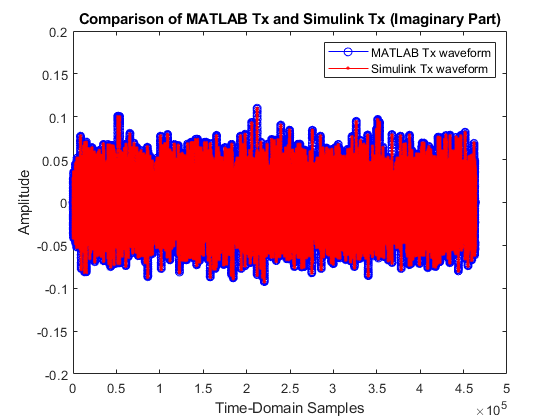# HDL OFDM MATLAB References

This example shows how to model OFDM transmitter, additive white Gaussian noise (AWGN), and OFDM receiver hardware algorithms in MATLAB® as steps toward developing a Simulink® implementation for hardware. The HDL OFDM MATLAB References example bridges the gap between a mathematical algorithm and its hardware implementation. This example provides MATLAB references of the HDL OFDM Transmitter, HDL AWGN, and HDL OFDM Receiver algorithms. You can use these MATLAB references to generate test vectors for verifying the HDL implementation of the HDL OFDM Transmitter, HDL Implementation of AWGN Generator, and HDL OFDM Receiver Simulink models.### HDL OFDM Transmitter MATLAB Reference

This section describes the MATLAB reference of HDL OFDM Transmitter.

This MATLAB reference accepts a modulation order, code rate index, number of frames, and data bits to be transmitted as a `txParam` structure or array of structures. `txParam` has these fields.

• `modOrder` — Specify 2, 4, 16, or 64 for 'BPSK', 'QPSK', '16QAM', and '64QAM', respectively. The default value is 4 ('QPSK').

• `codeRateIndex` — Specify 0, 1, 2, or 3 for the rates '1/2', '2/3', '3/4', and '5/6' respectively. The default value is 0 ('1/2').

• `numFrames` — Specify a positive integer. The default value is 5.

• `txDataBits` — Specify binary values in a row or column vector of length `trBlkSize` x `txParam.numFrames`. The default value is a column vector containing randomly generated binary values of length `trBlkSize` x `txParam.numFrames`.

Calculate the transport block size (`trBlkSize`) by using these parameters.

• `numSubCar` — Number of subcarriers per symbol

• `pilotsPerSym` — Number of pilots per symbol

• `numDataOFDMSymbols` — Number of data OFDM symbols

• `bitsPerModSym` — Number of bits per modulated symbol

• `codeRate` — Punctured code rate

• `dataConvK` — Constraint length of the convolutional encoder

• `dataCRCLen` — CRC length

`trBlkSize = ((numSubCar - pilotsPerSym) x numDataOFDMSymbols x bitsPerModSym x codeRate) - (dataConvK-1) - dataCRCLen`

For example, to generate a time-domain OFDM transmitter waveform of 5 frames with a modulation scheme of 16QAM and code rate of 1/2 using random data bits in the transport block, format the inputs as structure.

```txParam.modOrder = 16; % Modulation order corresponding to 16-QAM txParam.codeRateIndex = 0; % Code rate index corresponding to 1/2 txParam.numFrames = 5; % Number of frames to be generated % Calculate transport block size (trBlkSize) using parameters numSubCar = 72; % Number of subcarriers per symbol pilotsPerSym = 12; % Number of pilots per symbol numDataOFDMSymbols = 32; % Number of data OFDM symbols bitsPerModSym = log2(txParam.modOrder); % Bits per modulated symbol codeRate = 1/2; % Punctured code rate dataConvK = 7; % Constraint length of convolutional code polynomial dataCRCLen = 32; % Data CRC length trBlkSize = ((numSubCar-pilotsPerSym)*numDataOFDMSymbols* ... bitsPerModSym*codeRate) - (dataConvK-1) - dataCRCLen; txParam.txDataBits = randi([0 1],txParam.numFrames*trBlkSize,1); % Generate complex baseband transmitter waveform fprintf('\n-------------------------\n'); fprintf('\n Transmitting %d frames ...\n',txParam.numFrames); [txWaveform,txGrid,txDiagnostics] = whdlexamples.OFDMTx(txParam); fprintf('\n Transmission successful.\n'); fprintf('\n-------------------------\n'); ```
```------------------------- Transmitting 5 frames ... Transmission successful. ------------------------- ```

The `whdlexamples.OFDMTx` function returns arguments `txWaveform`, `txGrid`, and `txDiagnostics`.

• `txWaveform` is the generated time-domain waveform and is returned as a column vector of length ((`fftLen` + `cpLen`) x `txParam.numFrames` x `numSymPerFrame`), where:

1. `fftLen` is the FFT length.

2. `cpLen` is the cyclic prefix length.

3. `txParam.numFrames` is the number of OFDM frames generated.

4. `numSymPerFrame` is the number of OFDM symbols per frame.

If `txParam` is an array of structures, then in the expression `txParam.numFrames` is replaced with the sum of all `numFrames` attributes present in the array. The frame structure of the generated time-domain waveform `txWaveform` is similar to the Simulink HDL OFDM Transmitter output waveform. For the detailed explanation of the frame structure, see the HDL OFDM Transmitter example.

• `txGrid` is the transmitter grid output and is returned as a matrix of size `numSubCar`-by-(`txParam.numFrames` x `numSymPerFrame`), where `numSubCar` is the number of active subcarriers.

• `txDiagnostics` is a structure or array of structures and consists of these three fields:

1. `headerBits` represents the header bits as a column vector of size 14, which includes 3 bits for the FFT length index, 2 bits for the symbol modulation type, 2 bits for the code rate index, and 7 spare bits.

2. `dataBits` represents actual data bits transmitted in the given number of frames (`txParam.numFrames`). This field is a binary-valued row or column vector of length equal to (`txParam.numFrames` x `trbBlkSize`). Whether `dataBits` is a row or column vector depends on the dimension of `txParam.dataBits`. The default size is a column vector of length equal to `txParam.numFrames` x `trbBlkSize`.

3. `ofdmModOut` represents the OFDM modulator output as a column vector of length equal to (`fftLen` + `cpLen`) x `txParam.numFrames` x `numSymPerFrame`.

`OFDMTx`

`whdlexamples.OFDMTx` function is used to generate OFDM transmitter waveform with synchronization, reference, header, pilots, and data signals. This function returns `txWaveform`, `txGrid`, and `txDiagnostics` using transmitter parameters `txParam`. This function internally calls these individual functions.

• `generateOFDMSyncSignal` — This function generates the synchronization signal `SyncSignal`. This function uses Zadoff-Chu sequence with a root index of 25 and length of 62.

• `generateOFDMRefSignal` — This function generates the reference signal `refSignal` for the given FFT length `fftLen`. This function uses a BPSK-modulated pseudo random binary sequence.

• `generateOFDMPilotSignal` — This function generates the pilot signal `pilot`. This function uses a BPSK-modulated pseudo random binary sequence.

• `OFDMSymbolModulate` — This function modulates input bits to complex modulation symbols based on the specified modulation scheme BPSK, QPSK, 16QAM, and 64QAM.

Plot the resource grid of the transmitter waveform. The plot indicates the magnitude variations of each resource grid element.

```plotResourceGrid(txGrid); ```### HDL AWGN MATLAB Reference

This section describes the MATLAB reference of HDL AWGN.

This MATLAB reference is used for performance evaluation of the HDL OFDM Transmitter and Receiver algorithms. The HDL AWGN MATLAB reference generates AWGN by accepting the signal-to-noise ratio (SNR) in decibel (dB) and sets of seeds. For more details, see HDL Implementation of AWGN Generator. The generated AWGN is added to the HDL OFDM Transmitter output.

```FFTLen = 128; CPLen = 32; usedSubCarr = 72; % Out of 128 subcarriers, 72 subcarriers are loaded with data SNRdB = 30; SNRdBSimInput = SNRdB*ones(length(txWaveform)+633,1); seedsURNG1 = [121 719 511]; % Seeds for TausURNG1 seedsURNG2 = [2343 323 833]; % Seeds for TausURNG2 txScaleFactor = FFTLen/sqrt(usedSubCarr); awgnNoise = whdlexamples.hdlawgn(SNRdBSimInput,seedsURNG1,seedsURNG2); rxWaveform = txWaveform + (1/txScaleFactor)*awgnNoise(634:end); fprintf('\n Applying the AWGN channel at %d dB...\n', SNRdB); ```
``` Applying the AWGN channel at 30 dB... ```

### HDL OFDM Receiver MATLAB Reference

This section describes MATLAB reference of HDL OFDM Receiver.

This MATLAB reference includes time synchronization, CFO estimation and correction, OFDM demodulation, header recovery, CPE estimation and correction, and data recovery.

The `whdlexamples.OFDMRx` function accepts `rxWaveform`, a transmitted waveform passed through an AWGN channel.

The `whdlexamples.OFDMRx` function returns decoded bits `rxBits` and an array of structures, `rxDiagnostics`, consisting of these eight fields.

• `estCFO` — Estimated carrier frequency offset

• `rxConstellationHeader` — Demodulated header constellation symbols

• `rxConstellationData` — Demodulated data constellation symbols

• `softLLR` — Demodulated soft LLR bits

• `decodedCodeRateIndex` — Decoded code rate index from header

• `decodedModOrder` — Decoded modulation order from header

• `headerCRCErrorFlag` — Status of header CRC

• `dataCRCErrorFlag` — Status of data CRC

`OFDMRx`

The `whdlexamples.OFDMRx` function is used to demodulate and decode the received `rxWaveform`. This function internally calls these individual functions.

• `OFDMFrequencyOffset` — This function estimates the carrier frequency offset based on cyclic prefix (CP) technique. The cyclic prefix portion of the received time-domain waveform is correlated to estimate frequency offset.

• `OFDMFrequencyCorrect` — This function corrects the carrier frequency offset on the received waveform using the estimated frequency offset.

• `OFDMFrameSync` — This function synchronizes the received waveform by performing correlation using the reference signal. This step reduces the intersymbol interference while demodulating the received waveform.

• `OFDMDemodulation` — This function converts the time-domain waveform to frequency-domain waveform for further decoding. The object `dsp.HDLFFT` is used for HDL implementation of the receiver.

• `OFDMChannelEstimation` — This function performs the estimation of the channel using two reference signals. It uses least squares (LS) estimation technique. LS estimates are averaged to improve channel estimation accuracy.

• `OFDMChannelEqualization` — This function performs zero forcing (ZF) equalization using the estimated channel. Then the received waveform that is free of the channel is used for header recovery and data recovery.

• `OFDMHeaderRecovery` — This function recovers header information by performing symbol demodulation, deinterleaving, and Viterbi decoding. The CRC status indicates the success or failure of header information recovery. This header recovery CRC status is given as an output of the receiver to indicate frame loss or recovery. When the CRC check fails, the header CRC status is `1`. Otherwise, it is `0`.

• `OFDMDataRecovery` — This function performs symbol demodulation, deinterleaving, depuncturing, Viterbi decoding, and descrambling. The function processes the data only when the header CRC check passes. After descrambling the decoded data, CRC check is performed on the recovered data bits to indicate if the packet is errored. When the CRC check fails, the header CRC status is `1`. Otherwise, it is `0`.

```fprintf('\n Receiving process started...\n'); [rxDataBits,rxDiagnostics] = whdlexamples.OFDMRx(rxWaveform); fprintf('\n Reception completed\n\n'); % Plot constellation of header and data scatterplot(rxDiagnostics.rxConstellationHeader(:),1,0,'b.') title('Header Constellation') axisObj = gca; axisObj.XColor = 'w'; axisObj.YColor = 'w'; scatterplot(rxDiagnostics.rxConstellationData(:),1,0,'b.') title('Data Constellation'); axisObj = gca; axisObj.XColor = 'w'; axisObj.YColor = 'w'; ```
``` Receiving process started... Estimating carrier frequency offset ... First four frames are used for carrier frequency offset estimation. Estimated carrier frequency offset is -8.646138e-01 Hz. Detected and processing frame 5 ------------------------------------------ Header CRC passed Modulation: 16QAM, codeRate=1/2 and FFT Length=128 Data CRC passed Data decoding completed ------------------------------------------ Reception completed ```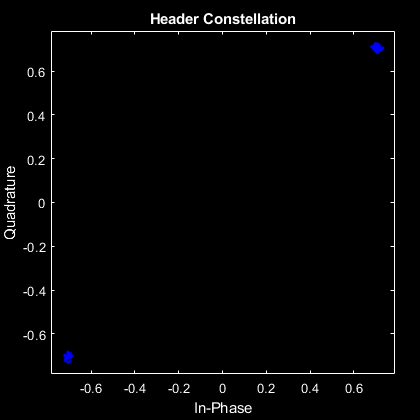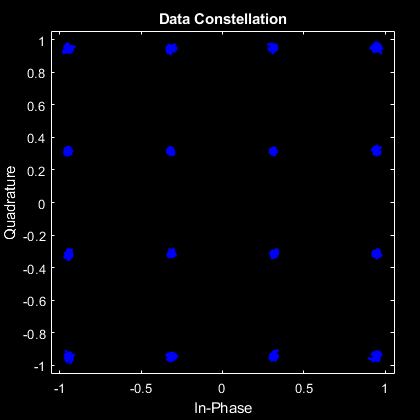### Verify Simulink Model with MATLAB Reference

In this section, the Simulink HDL OFDM Transmitter, AWGN generator, and Simulink HDL OFDM Receiver implemented in fixed point are compared with the equivalent MATLAB HDL reference models implemented in floating point.

The Simulink model consists of an OFDM Transmitter that generates a time-domain waveform for a user-defined modulation order and code rate. The time-domain waveform is then passed through the AWGN channel that introduces AWGN noise of the desired SNR in dB. Then, the OFDM Receiver is used to demodulate and decode information bits. The outputs of the Simulink model are verified with the MATLAB reference at each stage.

```open HDLOFDMTxRx; sim HDLOFDMTxRx; ```
```### Starting serial model reference simulation build ### Model reference simulation target for whdlOFDMRx is up to date. ### Model reference simulation target for whdlOFDMTx is up to date. Build Summary 0 of 2 models built (2 models already up to date) Build duration: 0h 0m 1.342s ```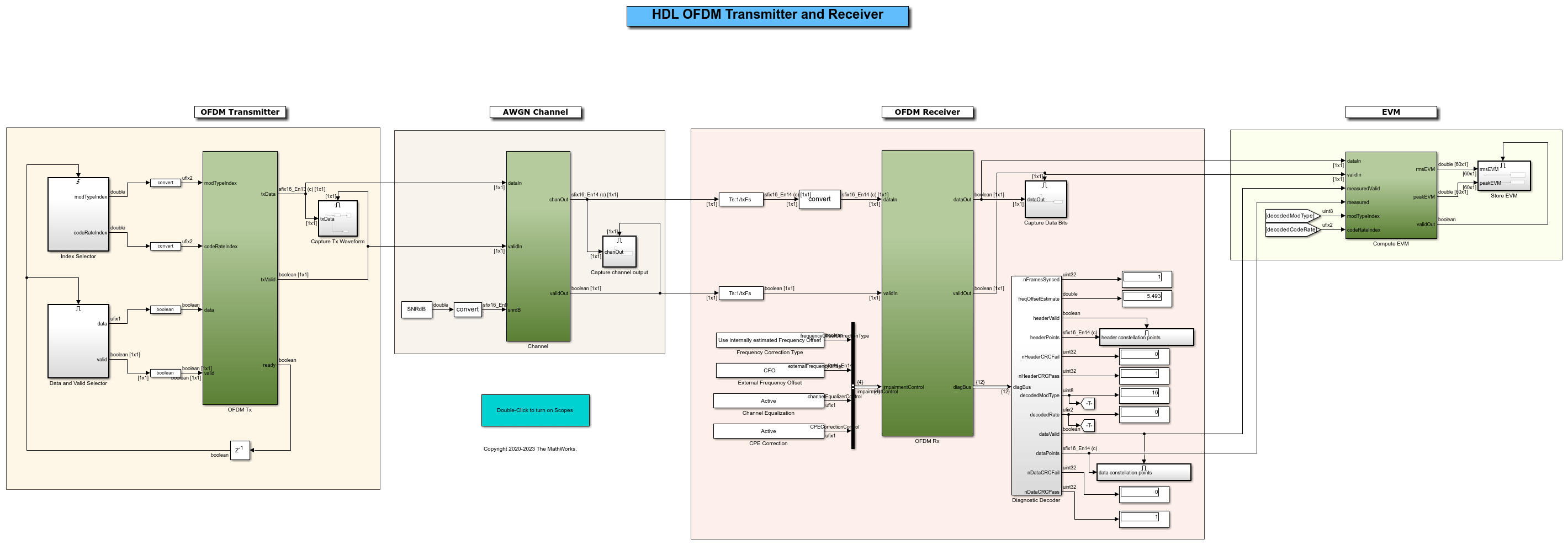Verify Simulink HDL OFDM Transmitter with MATLAB HDL OFDM Transmitter

In this section, plot the real and imaginary parts of the HDL OFDM Transmitter MATLAB reference function output `txWaveform` and compare with the output of the HDL OFDM Transmitter block.

```matlabTxWaveform = txWaveform; simulinkTxWaveform = simTxOut; figure; plot(real(matlabTxWaveform),'-bo') hold on plot(real(simulinkTxWaveform(1:length(matlabTxWaveform))),'-r.') legend('MATLAB Tx waveform','Simulink Tx waveform'); title('Comparison of MATLAB Tx and Simulink Tx (Real Part)'); ylim([-0.2 0.2]); xlabel('Time-Domain Samples'); ylabel('Amplitude'); figure; plot(imag(matlabTxWaveform),'-bo') hold on plot(imag(simulinkTxWaveform(1:length(matlabTxWaveform))),'-r.') legend('MATLAB Tx waveform','Simulink Tx waveform'); title('Comparison of MATLAB Tx and Simulink Tx (Imaginary Part)'); ylim([-0.2 0.2]); xlabel('Time-Domain Samples'); ylabel('Amplitude'); ```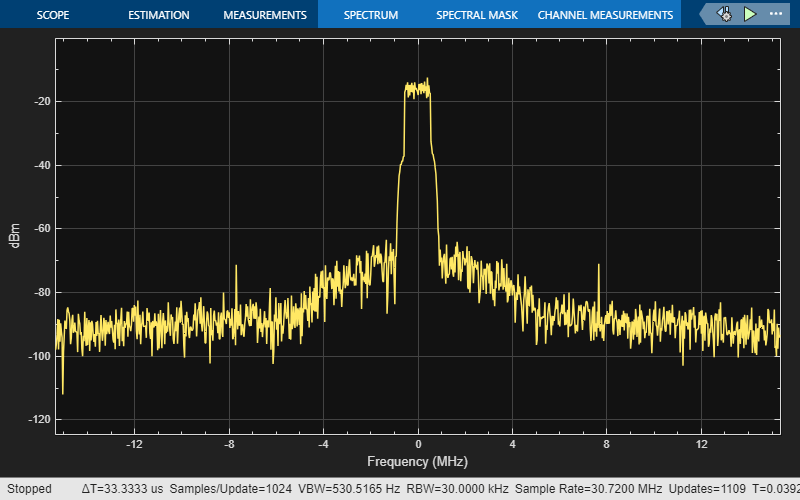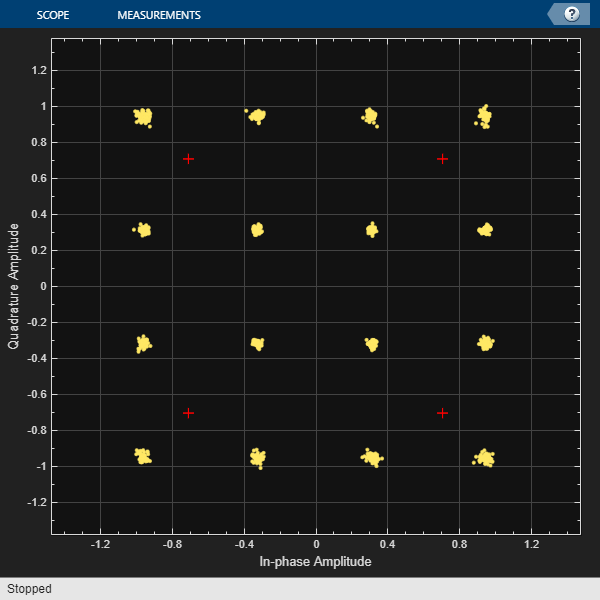Verify Simulink HDL AWGN Generator with MATLAB HDL AWGN

In this section, plot the real and imaginary parts of the MATLAB HDL AWGN is compared with the output of the Simulink AWGN Generator block.

```matlabChannelOut= rxWaveform; simulinkChannelOut = simChannelOut; figure; plot(real(matlabChannelOut),'-bo'); hold on; plot(real(simulinkChannelOut(1:length(matlabChannelOut))),'-r.'); legend('MATLAB channel output','Simulink channel output'); title('Comparison of MATLAB Channel and Simulink Channel (Real Part)'); ylim([-0.2 0.2]); xlabel('Time-Domain Samples'); ylabel('Amplitude'); figure; plot(imag(matlabChannelOut),'-bo'); hold on; plot(imag(simulinkChannelOut(1:length(matlabChannelOut))),'-r.'); legend('MATLAB channel output','Simulink channel output'); title('Comparison of MATLAB Channel and Simulink Channel (Imaginary Part)'); ylim([-0.2 0.2]); xlabel('Time-Domain Samples'); ylabel('Amplitude'); ```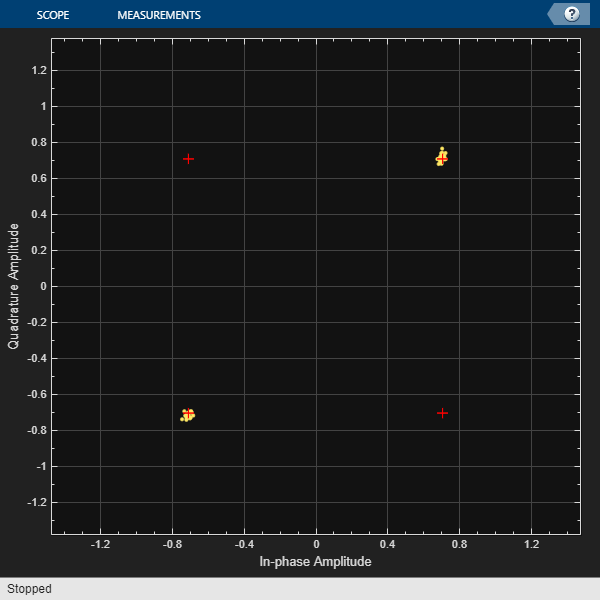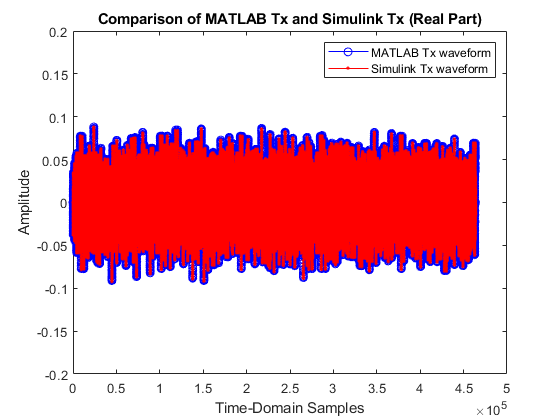In this section, plot the decoded bits of the MATLAB receiver as compared with the decoded bits of the Simulink receiver.

```matlabRxOut= rxDataBits; simulinkRxOut = simRxDataBits; figure; plot(rxDataBits,'-bo'); hold on; plot(simulinkRxOut(1:length(rxDataBits)),'-r.'); legend('MATLAB Rx bits','Simulink Rx bits'); title('MATLAB and Simulink Decoded Bits'); ylim([-0.25 1.25]); xlabel('Time-domain Samples'); ylabel('Amplitude'); ```